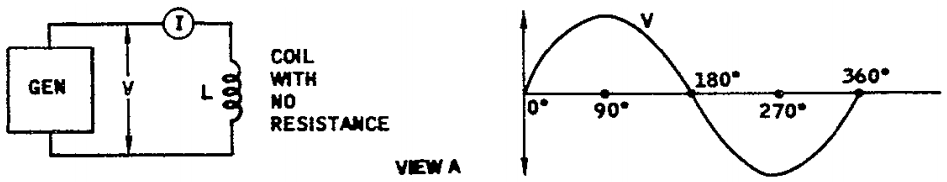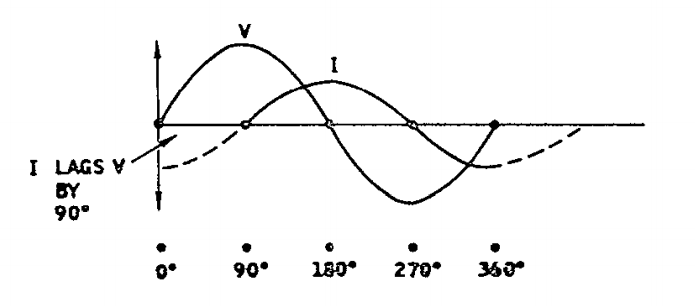PB_00 - Capítulo 5 - Análise de Fase - Página 5-034

Certainly true. When a resistor is connected across the generator, the current will be in phase with the voltage.Inductance has a unique property that opposes a change in current. For example, if a sudden change in voltage is applied to a coil, the current will not immediately change.

Instead, it lags the voltage. To get a better feel for this, visualize that the voltage rises above and below a center value as shown in view A. To give us a time base, let's use a circle with 360 degrees. Then let's agree that the voltage first rises to a maximum value in one direction. At this point we have used 90 degrees of "our time."

Now let the voltage fall to the center value (180 degrees) and rise to a maximum value in the opposite direction (270 degrees). And finally let's let the voltage return to the center value (360 degrees). Visualize that this 360 degree cycle is repeated again and again. The result is an alternating voltage.

If we have a means of measuring how the current (I) varies as the voltage (V) varies, and if we plot this on our time base (360 degrees), we get the following result. This shows us that the current (I) is lagging the voltage (V) by 90 degrees. Keep in mind that the sequence from 0 degrees to 360 degrees is time. Note that as the voltage rises to a maximum at 90 degrees, the current is decreasing to the center value.